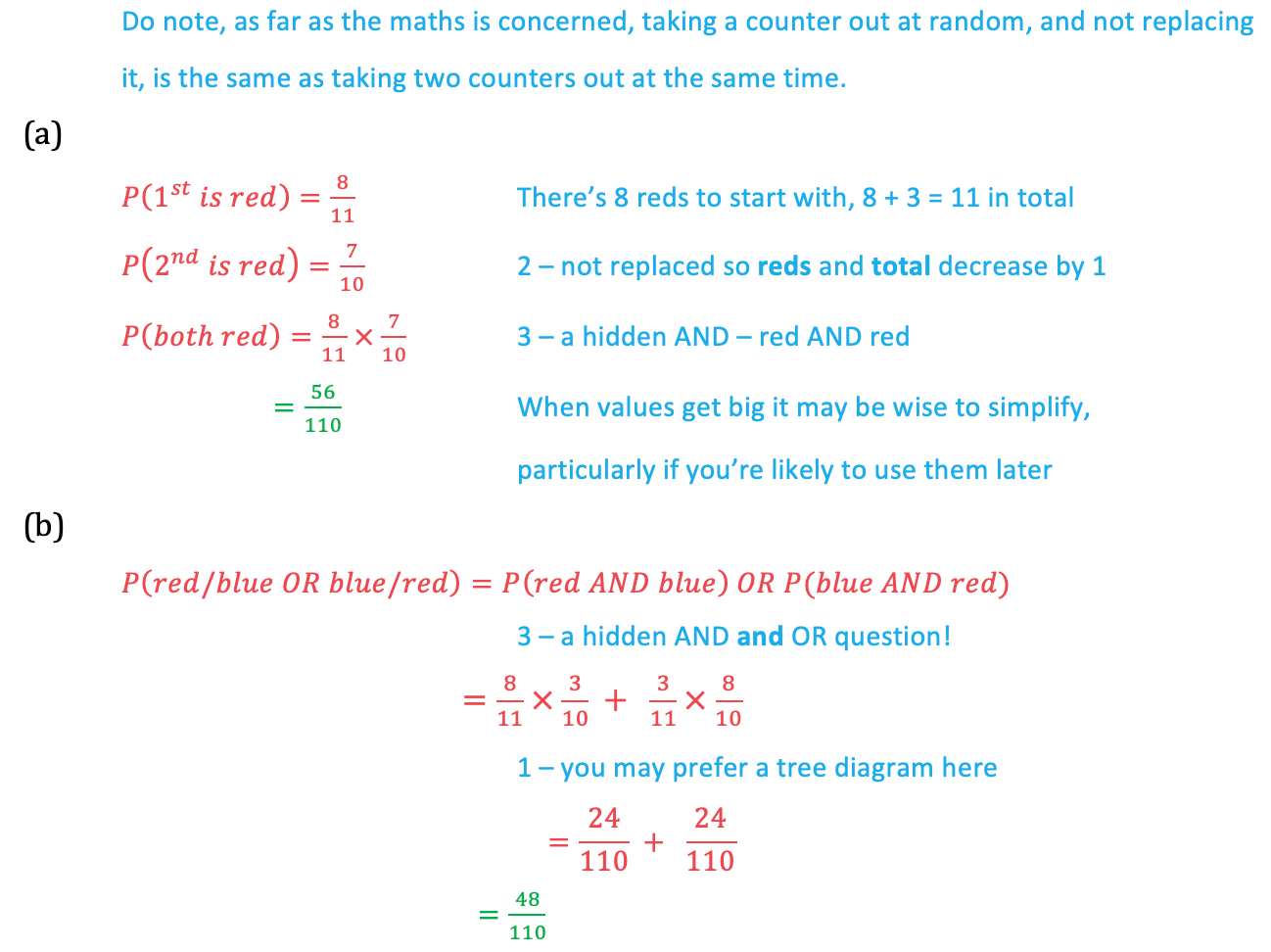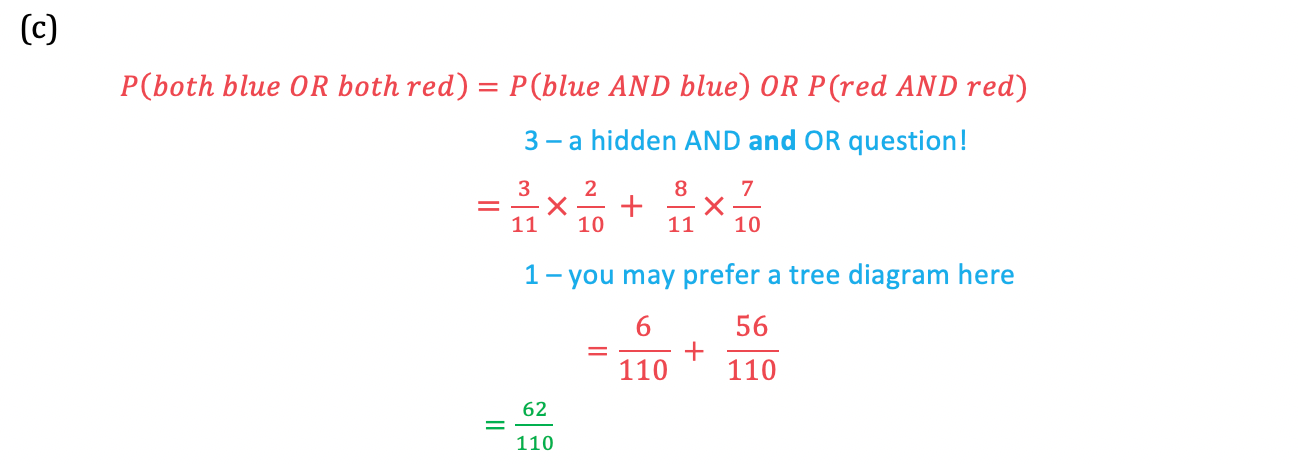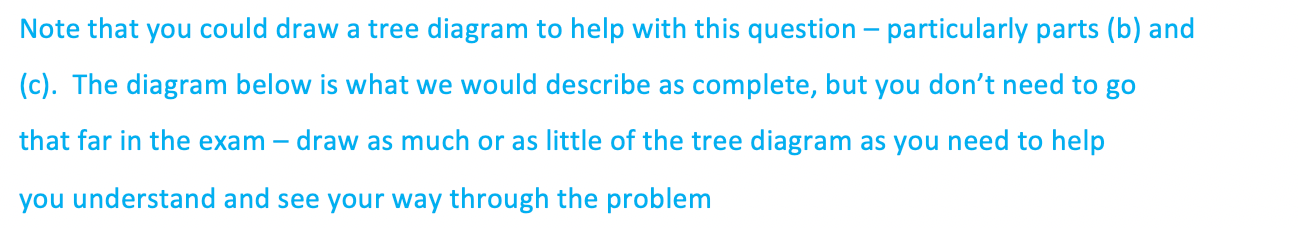# Edexcel IGCSE Maths 复习笔记 6.6.1 Combined Probability - Basics

Edexcel IGCSE Maths 复习笔记 6.6.1 Combined Probability - Basics

#### What do we mean by combined probabilities?

• This can mean lots of things as you’ll see over these notes and the next set
• In general it means we have more than one ‘thing’ (trial/event) to bear in mind and these things may be independentmutually exclusive or may involve an event that follows on from a previous event drawing a second counter from a bag

1. Tree diagrams

• Especially useful when we have more than one trial but are only concerned with two outcomes from each
• Even more useful when probabilities change for the second experiment

2. Replacement

• Are items being selected at random replaced or not?
• If not then numbers will decrease as the situation progresses and so probabilities change – this is often called conditional probability

3. AND’s and OR’s

• AND means for independent events
• OR means for mutually exclusive events

4. Sum of all probabilities is 1

• This is a very basic fact that gets lost along the way in more complicated probability questions – but it is one of the best ‘tricks’ you can use!
• A good example of its use is when you want the probability of something being “non zero”:P(x ≥ 1) = 1 - P(x = 0)

#### Worked Example#### Worked Example#### Worked Example#### Worked Example